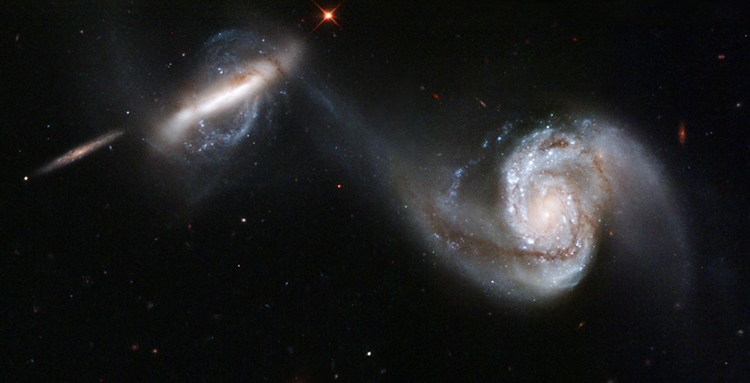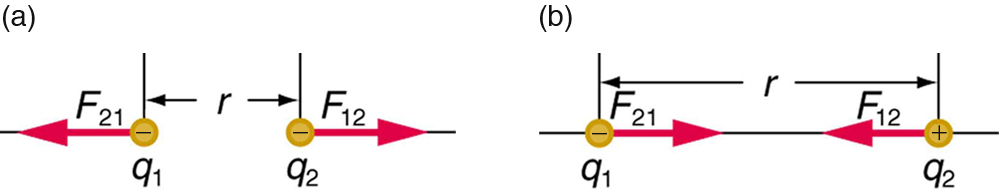# 29.1 Coulomb’s law

 Page 1 / 6
• State Coulomb’s law in terms of how the electrostatic force changes with the distance between two objects.
• Calculate the electrostatic force between two charged point forces, such as electrons or protons.
• Compare the electrostatic force to the gravitational attraction for a proton and an electron; for a human and the Earth.This NASA image of Arp 87 shows the result of a strong gravitational attraction between two galaxies. In contrast, at the subatomic level, the electrostatic attraction between two objects, such as an electron and a proton, is far greater than their mutual attraction due to gravity. (credit: NASA/HST)

Through the work of scientists in the late 18th century, the main features of the electrostatic force    —the existence of two types of charge, the observation that like charges repel, unlike charges attract, and the decrease of force with distance—were eventually refined, and expressed as a mathematical formula. The mathematical formula for the electrostatic force is called Coulomb’s law    after the French physicist Charles Coulomb (1736–1806), who performed experiments and first proposed a formula to calculate it.

## Coulomb’s law

$F=k\frac{|{q}_{1}{q}_{2}|}{{r}^{2}}.$

Coulomb’s law calculates the magnitude of the force $F$ between two point charges, ${q}_{1}$ and ${q}_{2}$ , separated by a distance $r$ . In SI units, the constant $k$ is equal to

$k=8\text{.}\text{988}×{\text{10}}^{9}\frac{\text{N}\cdot {\text{m}}^{2}}{{\text{C}}^{2}}\approx 8\text{.}\text{99}×{\text{10}}^{9}\frac{\text{N}\cdot {\text{m}}^{2}}{{\text{C}}^{2}}.$

The electrostatic force is a vector quantity and is expressed in units of newtons. The force is understood to be along the line joining the two charges. (See [link] .)

Although the formula for Coulomb’s law is simple, it was no mean task to prove it. The experiments Coulomb did, with the primitive equipment then available, were difficult. Modern experiments have verified Coulomb’s law to great precision. For example, it has been shown that the force is inversely proportional to distance between two objects squared $\left(F\propto 1/{r}^{2}\right)$ to an accuracy of 1 part in ${\text{10}}^{\text{16}}$ . No exceptions have ever been found, even at the small distances within the atom.The magnitude of the electrostatic force F size 12{F} {} between point charges q 1 size 12{q rSub { size 8{1} } } {} and q 2 size 12{q rSub { size 8{2} } } {} separated by a distance r size 12{F} {} is given by Coulomb’s law. Note that Newton’s third law (every force exerted creates an equal and opposite force) applies as usual—the force on q 1 size 12{q rSub { size 8{1} } } {} is equal in magnitude and opposite in direction to the force it exerts on q 2 size 12{q rSub { size 8{2} } } {} . (a) Like charges. (b) Unlike charges.

## How strong is the coulomb force relative to the gravitational force?

Compare the electrostatic force between an electron and proton separated by $0\text{.}\text{530}×{\text{10}}^{-\text{10}}\phantom{\rule{0.25em}{0ex}}\text{m}$ with the gravitational force between them. This distance is their average separation in a hydrogen atom.

Strategy

To compare the two forces, we first compute the electrostatic force using Coulomb’s law, $F=k\frac{|{q}_{1}{q}_{2}|}{{r}^{2}}$ . We then calculate the gravitational force using Newton’s universal law of gravitation. Finally, we take a ratio to see how the forces compare in magnitude.

Solution

Entering the given and known information about the charges and separation of the electron and proton into the expression of Coulomb’s law yields

$F=k\frac{|{q}_{1}{q}_{2}|}{{r}^{2}}$
$\begin{array}{}=\left(8.99×{\text{10}}^{9}\phantom{\rule{0.25em}{0ex}}\text{N}\cdot {\text{m}}^{2}/{\text{C}}^{2}\right)×\frac{\left(\text{1.60}×{\text{10}}^{\text{–19}}\phantom{\rule{0.25em}{0ex}}\text{C}\right)\left(1.60×{\text{10}}^{\text{–19}}\phantom{\rule{0.25em}{0ex}}\text{C}\right)}{\left(0.530×{\text{10}}^{\text{–10}}\phantom{\rule{0.25em}{0ex}}\text{m}{\right)}^{2}}\\ \end{array}$

Thus the Coulomb force is

$F=\text{8.19}×{\text{10}}^{\text{–8}}\phantom{\rule{0.25em}{0ex}}\text{N}.$

The charges are opposite in sign, so this is an attractive force. This is a very large force for an electron—it would cause an acceleration of $8.99×{\text{10}}^{\text{22}}\phantom{\rule{0.25em}{0ex}}\text{m}/{\text{s}}^{2}$ (verification is left as an end-of-section problem).The gravitational force is given by Newton’s law of gravitation as:

${F}_{G}=G\frac{\mathrm{mM}}{{r}^{2}},$

where $G=6.67×{\text{10}}^{-\text{11}}\phantom{\rule{0.25em}{0ex}}\text{N}\cdot {\text{m}}^{2}/{\text{kg}}^{2}$ . Here $m$ and $M$ represent the electron and proton masses, which can be found in the appendices. Entering values for the knowns yields

${F}_{G}=\left(6.67×{\text{10}}^{–\text{11}}\phantom{\rule{0.25em}{0ex}}\text{N}\cdot {\text{m}}^{2}/{\text{kg}}^{2}\right)×\frac{\left(9.11×{\text{10}}^{\text{–31}}\phantom{\rule{0.25em}{0ex}}\text{kg}\right)\left(1.67×{\text{10}}^{\text{–27}}\phantom{\rule{0.25em}{0ex}}\text{kg}\right)}{\left(0.530×{\text{10}}^{\text{–10}}\phantom{\rule{0.25em}{0ex}}\text{m}{\right)}^{2}}=3.61×{\text{10}}^{\text{–47}}\phantom{\rule{0.25em}{0ex}}\text{N}$

This is also an attractive force, although it is traditionally shown as positive since gravitational force is always attractive. The ratio of the magnitude of the electrostatic force to gravitational force in this case is, thus,

$\frac{F}{{F}_{G}}=\text{2}\text{.}\text{27}×{\text{10}}^{\text{39}}.$

Discussion

This is a remarkably large ratio! Note that this will be the ratio of electrostatic force to gravitational force for an electron and a proton at any distance (taking the ratio before entering numerical values shows that the distance cancels). This ratio gives some indication of just how much larger the Coulomb force is than the gravitational force between two of the most common particles in nature.

Is there any normative that regulates the use of silver nanoparticles?
what king of growth are you checking .?
Renato
What fields keep nano created devices from performing or assimulating ? Magnetic fields ? Are do they assimilate ?
why we need to study biomolecules, molecular biology in nanotechnology?
?
Kyle
yes I'm doing my masters in nanotechnology, we are being studying all these domains as well..
why?
what school?
Kyle
biomolecules are e building blocks of every organics and inorganic materials.
Joe
anyone know any internet site where one can find nanotechnology papers?
research.net
kanaga
sciencedirect big data base
Ernesto
Introduction about quantum dots in nanotechnology
what does nano mean?
nano basically means 10^(-9). nanometer is a unit to measure length.
Bharti
do you think it's worthwhile in the long term to study the effects and possibilities of nanotechnology on viral treatment?
absolutely yes
Daniel
how to know photocatalytic properties of tio2 nanoparticles...what to do now
it is a goid question and i want to know the answer as well
Maciej
Abigail
for teaching engĺish at school how nano technology help us
Anassong
Do somebody tell me a best nano engineering book for beginners?
there is no specific books for beginners but there is book called principle of nanotechnology
NANO
what is fullerene does it is used to make bukky balls
are you nano engineer ?
s.
fullerene is a bucky ball aka Carbon 60 molecule. It was name by the architect Fuller. He design the geodesic dome. it resembles a soccer ball.
Tarell
what is the actual application of fullerenes nowadays?
Damian
That is a great question Damian. best way to answer that question is to Google it. there are hundreds of applications for buck minister fullerenes, from medical to aerospace. you can also find plenty of research papers that will give you great detail on the potential applications of fullerenes.
Tarell
what is the Synthesis, properties,and applications of carbon nano chemistry
Mostly, they use nano carbon for electronics and for materials to be strengthened.
Virgil
is Bucky paper clear?
CYNTHIA
carbon nanotubes has various application in fuel cells membrane, current research on cancer drug,and in electronics MEMS and NEMS etc
NANO
so some one know about replacing silicon atom with phosphorous in semiconductors device?
Yeah, it is a pain to say the least. You basically have to heat the substarte up to around 1000 degrees celcius then pass phosphene gas over top of it, which is explosive and toxic by the way, under very low pressure.
Harper
Do you know which machine is used to that process?
s.
how to fabricate graphene ink ?
for screen printed electrodes ?
SUYASH
What is lattice structure?
of graphene you mean?
Ebrahim
or in general
Ebrahim
in general
s.
Graphene has a hexagonal structure
tahir
On having this app for quite a bit time, Haven't realised there's a chat room in it.
Cied
what is biological synthesis of nanoparticles
Got questions? Join the online conversation and get instant answers!By Qqq QqqByByBy